Test: Complex Variables

# Test: Complex Variables

Test Description

## 15 Questions MCQ Test GATE Electrical Engineering (EE) 2023 Mock Test Series | Test: Complex Variables

Test: Complex Variables for Electronics and Communication Engineering (ECE) 2023 is part of GATE Electrical Engineering (EE) 2023 Mock Test Series preparation. The Test: Complex Variables questions and answers have been prepared according to the Electronics and Communication Engineering (ECE) exam syllabus.The Test: Complex Variables MCQs are made for Electronics and Communication Engineering (ECE) 2023 Exam. Find important definitions, questions, notes, meanings, examples, exercises, MCQs and online tests for Test: Complex Variables below.
Solutions of Test: Complex Variables questions in English are available as part of our GATE Electrical Engineering (EE) 2023 Mock Test Series for Electronics and Communication Engineering (ECE) & Test: Complex Variables solutions in Hindi for GATE Electrical Engineering (EE) 2023 Mock Test Series course. Download more important topics, notes, lectures and mock test series for Electronics and Communication Engineering (ECE) Exam by signing up for free. Attempt Test: Complex Variables | 15 questions in 45 minutes | Mock test for Electronics and Communication Engineering (ECE) preparation | Free important questions MCQ to study GATE Electrical Engineering (EE) 2023 Mock Test Series for Electronics and Communication Engineering (ECE) Exam | Download free PDF with solutions
 1 Crore+ students have signed up on EduRev. Have you?
Test: Complex Variables - Question 1

### The integration of f (z) = x2 + ixy from A(1, 1) to B(2, 4) along the straight line AB joining the two points is

Detailed Solution for Test: Complex Variables - Question 1

x = at + b, y = ct + d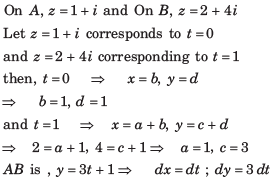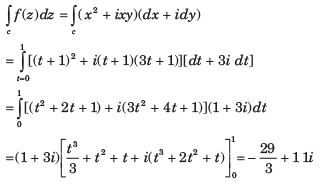Test: Complex Variables - Question 2

###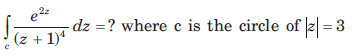​ ​ ​

Detailed Solution for Test: Complex Variables - Question 2

We know by the derivative of an analytic function that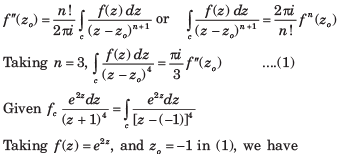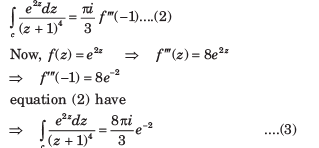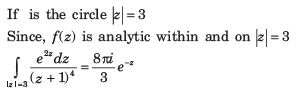Test: Complex Variables - Question 3

###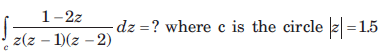Detailed Solution for Test: Complex Variables - Question 3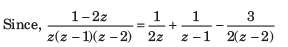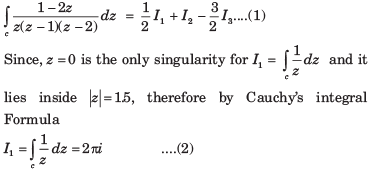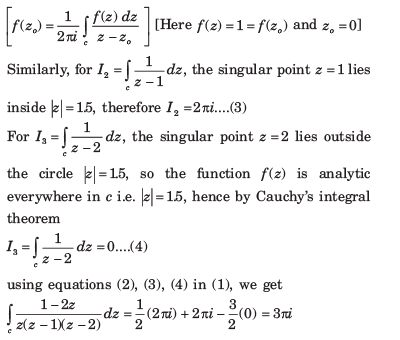Test: Complex Variables - Question 4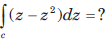where c is the upper half of the circle z = 1

Detailed Solution for Test: Complex Variables - Question 4

Given contour c is the circle |z| = 1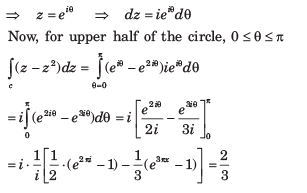Test: Complex Variables - Question 5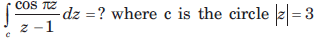Detailed Solution for Test: Complex Variables - Question 5

Let f (z) = cosπz then f(z) is analytic within and on |z| =3, now by Cauchy’s integral formula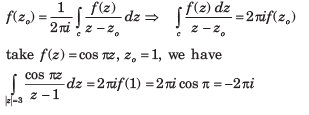Test: Complex Variables - Question 6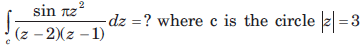Detailed Solution for Test: Complex Variables - Question 6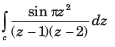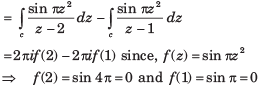Test: Complex Variables - Question 7

The value of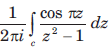around a rectangle with vertices at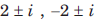is

Detailed Solution for Test: Complex Variables - Question 7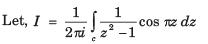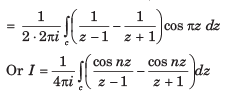Test: Complex Variables - Question 8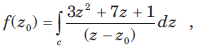where c is the circle x2 + y2 = 4

Que: The value of f(3) is

Detailed Solution for Test: Complex Variables - Question 8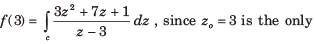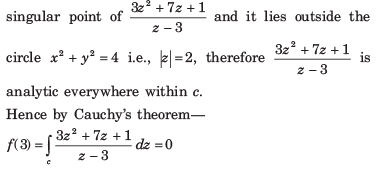Test: Complex Variables - Question 9where c is the circle x2 + y2 = 4

Que: The value of f' (1 - i) is

Detailed Solution for Test: Complex Variables - Question 9

The point (1 - i) lies within circle |z| = 2 ( ... the distance of 1 - i i.e., (1, 1) from the origin is √2 which is less than 2, the radius of the circle).

Let Ø(z) = 3z2 + 7z + 1 then by Cauchy’s integral formula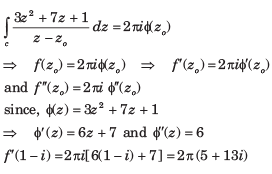Test: Complex Variables - Question 10

Expand the given function in Taylor’s series.

Que: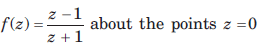Detailed Solution for Test: Complex Variables - Question 10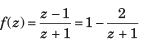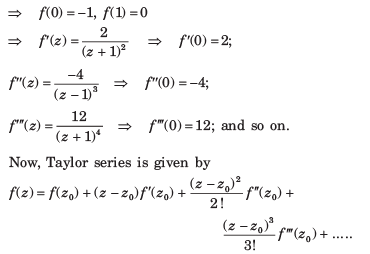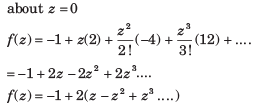Test: Complex Variables - Question 11

Expand the given function in Taylor’s series

Que: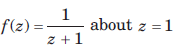Detailed Solution for Test: Complex Variables - Question 11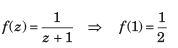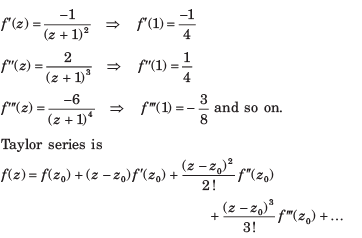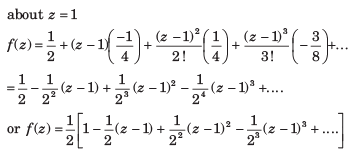Test: Complex Variables - Question 12

Expand the given function in Taylor’s series.

Que: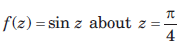Detailed Solution for Test: Complex Variables - Question 12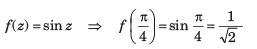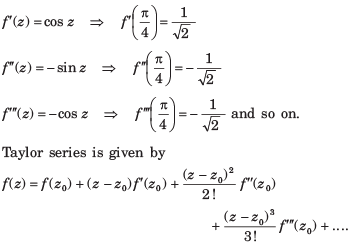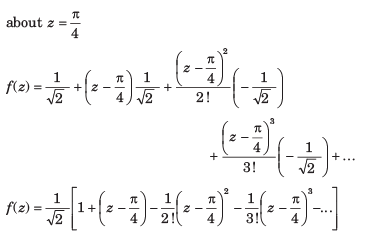Test: Complex Variables - Question 13

If |z + 1|  < 1, then z-2 is equal to

Detailed Solution for Test: Complex Variables - Question 13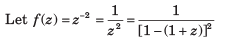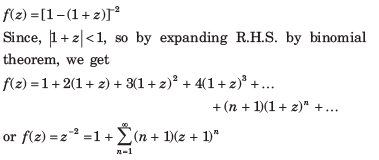Test: Complex Variables - Question 14

Expand the function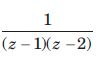in Laurent’s series for the condition given in question.

Que: 1 < |z| < 2

Detailed Solution for Test: Complex Variables - Question 14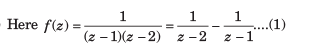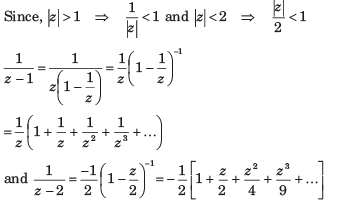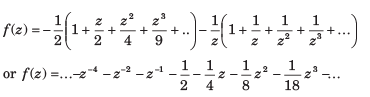Test: Complex Variables - Question 15

Expand the functionin Laurent’s series for the condition given in question.

Que: |z| > 2

Detailed Solution for Test: Complex Variables - Question 15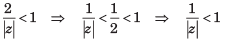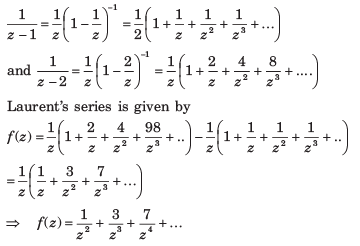## GATE Electrical Engineering (EE) 2023 Mock Test Series

22 docs|274 tests
 Use Code STAYHOME200 and get INR 200 additional OFF Use Coupon Code
Information about Test: Complex Variables Page
In this test you can find the Exam questions for Test: Complex Variables solved & explained in the simplest way possible. Besides giving Questions and answers for Test: Complex Variables, EduRev gives you an ample number of Online tests for practice

## GATE Electrical Engineering (EE) 2023 Mock Test Series

22 docs|274 tests